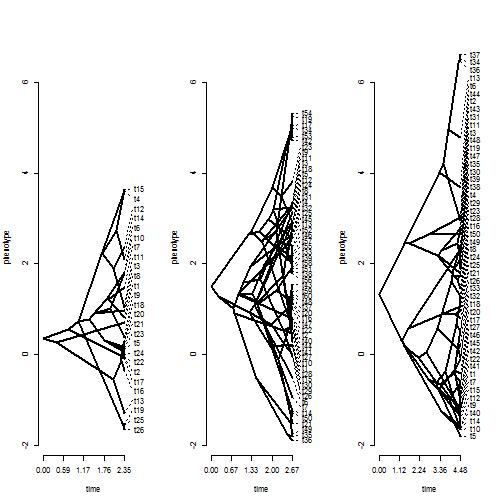## Wednesday, February 1, 2017

### Testing whether the rate of evolution of a continuous trait is different in different trees

I was recently asked by a colleague if I could help develop a method to test if the rate of evolution of a continuous character was the same or different in different trees.

This is closely related to the censored approach to test for changes in the rate of evolution developed by Brian O'Meara & colleagues in 2006. (Note that this should also be related to a method published by Dean Adams in 2013.) Here, though, rather than supply a single tree & specifying rate regimes within that tree, we can supply multiple trees (as an object of class `"multiPhylo"`) and a list of corresponding vectors of trait values for each tree.

First, here is our function:

``````ratebytree<-function(trees,x,...){
N<-length(trees)
## first, fit multi-rate model
lik.multi<-function(theta,trees,x){
n<-sapply(trees,Ntip)
N<-length(trees)
sig<-theta[1:N]
a<-theta[(N+1):(2*N)]
C<-lapply(trees,vcv)
V<-mapply("*",C,sig)
logL<-0
for(i in 1:N)
logL<-logL-t(x[[i]]-a[i])%*%solve(V[[i]])%*%(x[[i]]-
a[i])/2-n[i]*log(2*pi)/2-determinant(V[[i]])\$modulus/2
-logL
}
foo<-function(tree,x){
obj<-phyl.vcv(as.matrix(x),vcv(tree),1)
c(obj\$R[1,1],obj\$a[1,1])
}
obj<-mapply(foo,trees,x)
p<-as.vector(t(obj))
fit.multi<-optim(p,lik.multi,trees=trees,x=x,method="L-BFGS-B",
lower=c(rep(0,N),rep(-Inf,N)),upper=c(rep(Inf,N),rep(Inf,N)))
## now fit single-rate model
lik.onerate<-function(theta,trees,x){
n<-sapply(trees,Ntip)
N<-length(trees)
sig<-theta
a<-theta[1:N+1]
C<-lapply(trees,vcv)
V<-lapply(C,"*",sig)
logL<-0
for(i in 1:N)
logL<-logL-t(x[[i]]-a[i])%*%solve(V[[i]])%*%(x[[i]]-
a[i])/2-n[i]*log(2*pi)/2-determinant(V[[i]])\$modulus/2
-logL
}
p<-c(mean(fit.multi\$par[1:N]),fit.multi\$par[(N+1):(2*N)])
fit.onerate<-optim(p,lik.onerate,trees=trees,x=x,method="L-BFGS-B",
lower=c(0,rep(-Inf,N)),upper=c(Inf,rep(Inf,N)))
## compare models:
LR<-2*(-fit.multi\$value+fit.onerate\$value)
P.chisq<-pchisq(LR,df=N-1,lower.tail=FALSE)
obj<-list(
multi.rate.model=list(sig2=fit.multi\$par[1:N],
a=fit.multi\$par[(N+1):(2*N)],
k=2*N,
logL=-fit.multi\$value,
counts=fit.multi\$counts,convergence=fit.multi\$convergence,
message=fit.multi\$message),
common.rate.model=list(sig2=fit.onerate\$par,
a=fit.onerate\$par[1:N+1],
k=N+1,
logL=-fit.onerate\$value,
counts=fit.onerate\$counts,convergence=fit.onerate\$convergence,
message=fit.onerate\$message),
N=N,likelihood.ratio=LR,P.chisq=P.chisq)
class(obj)<-"ratebytree"
obj
}

## S3 print method
print.ratebytree<-function(x,...){
if(hasArg(digits)) digits<-list(...)\$digits
else digits<-4
N<-x\$N
cat("ML common-rate model:\n")
cat(paste("\ts^2\t",paste(paste("a[",1:N,"]",sep=""),collapse="\t")),
"\tk\tlogL\n")
cat(paste("value",round(x\$common.rate.model\$sig2,digits),
paste(round(x\$common.rate.model\$a,digits),collapse="\t"),
x\$common.rate.model\$k,round(x\$common.rate.model\$logL,digits),
"\n\n",sep="\t"))
cat("ML multi-rate model:\n")
cat(paste("\t",paste(paste("s^2[",1:N,"]",sep=""),collapse="\t"),"\t",
paste(paste("a[",1:N,"]",sep=""),collapse="\t")),
"\tk\tlogL\n")
cat(paste("value",paste(round(x\$multi.rate.model\$sig2,digits),collapse="\t"),
paste(round(x\$multi.rate.model\$a,digits),collapse="\t"),
x\$multi.rate.model\$k,round(x\$multi.rate.model\$logL,digits),
"\n\n",sep="\t"))
cat(paste("Likelihood ratio:",round(x\$likelihood.ratio,digits),"\n"))
cat(paste("P-value (based on X^2):",round(x\$P.chisq,digits),"\n\n"))
if(x\$multi.rate.model\$convergence==0&&x\$common.rate.model\$convergence==0)
cat("R thinks it has found the ML solution.\n\n")
else cat("One or the other optimization may not have converged.\n\n")
}
``````

Now let's try it with some simulated data.

First, here's a visualization of our data:

``````ylim<-range(c(x,y,z))
par(mfrow=c(1,3))
``````
``````## Optimizing the positions of the tip labels...
``````
``````phenogram(t3,z,ylim=ylim,spread.cost=c(1,0))
``````
``````## Optimizing the positions of the tip labels...
``````It's hard to tell from this whether the rates of each clade are the same or different - especially because the trees all have different total depths.

Now we're ready to fit our models

``````library(phytools)
fit<-ratebytree(c(t1,t2,t3),list(x,y,z))
fit
``````
``````## ML common-rate model:
##  s^2  a   a    a    k   logL
## value    1.4387  0.3648  1.5135  1.3195  4   -189.3164
##
## ML multi-rate model:
##   s^2 s^2  s^2   a   a    a    k   logL
## value    1.3265  1.9868  0.8394  0.3648  1.5135  1.3195  6   -184.4735
##
## Likelihood ratio: 9.6859
## P-value (based on X^2): 0.0079
##
## R thinks it has found the ML solution.
``````

Note that tree #1 and tree #3 have very comparable estimated rates (actually tree #1 is a little higher), even though the range of values is substantially greater on tree #3. Neat.

For the record, these data were simulated as follows:

``````t1<-pbtree(n=26)
t2<-pbtree(n=60)
t3<-pbtree(n=50)
x<-fastBM(t1,sig2=1)
y<-fastBM(t2,sig2=2)
z<-fastBM(t3,sig2=1)
``````

#### 1 comment:

1.This function is now in phytools. I recommend install phytools from GitHub using devtools rather than using the version posted above.

Note: due to the very large amount of spam, all comments are now automatically submitted for moderation.